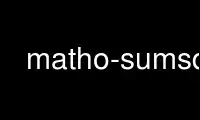# matho-sumsq - Online in the CloudThis is the command matho-sumsq that can be run in the OnWorks free hosting provider using one of our multiple free online workstations such as Ubuntu Online, Fedora Online, Windows online emulator or MAC OS online emulator

### PROGRAM:

#### NAME

matho-sumsq - Find the minimum sum of the squares for integers

#### SYNOPSIS

matho-sumsq [numbers]

#### DESCRIPTION

This command-line utility is optionally part of the mathomatic(1) package. It finds the
minimum number of positive integers that when squared and added together, equal the given
number. There is a proof that no more than 4 squares summed together are required to
represent any positive integer.

The command-line may contain positive integers to find the minimum squares of, they must
be less than 2147483648 (2^31) on 32-bit systems or less than 9223372036854775808 (2^63)
on 64-bit systems. If "+" is appended to the given number, the program counts up from the
given number. If the minimum number of squares is 2, this program displays all possible
combinations with 2 squares for the given number, otherwise it just displays the first
combination it finds.

If no command-line arguments are given, the programs reads the numbers from standard
input.

Use matho-sumsq online using onworks.net services

Free Servers & Workstations